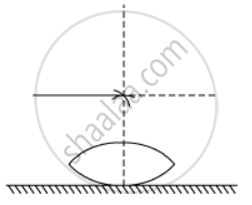# A Symmetric Biconvex Lens of the Radius of Curvature R and Made of Glass of Refractive Index 1.5, is Placed on a Layer of Liquid Placed on Top of a Plane Mirror as Shown in the Figure on Removing the Liquid Layer and Repeating the Experiment, the Distance is Found to Be Y. Obtain the Expression for the Refractive Index of the Liquid in Terms of X and Y - Physics

A symmetric biconvex lens of the radius of curvature R and made of glass of refractive index 1.5, is placed on a layer of liquid placed on top of a plane mirror as shown in the figure. An optical needle with its tip on the principal axis of the lens is moved along the axis until its real, inverted image coincides with the needle itself. The distance of the needle from the lens is measured to be x. On removing the liquid layer and repeating the experiment, the distance is found to be y. Obtain the expression for the refractive index of the liquid in terms of x and y#### Solution

Using the Lens makers formula, we know that

1/f_1 = (mu_"lens" - 1) [1/R - (-1/R)]

1/f_1  = (1.5 - 1) [2/R]

or f_1 = R

Similary for the other face of the lens we can write,

1/f_2  = (mu_"liquid" - 1)[1/(-R) - (1/oo)]

1/f_2  = (mu - 1) [-1/R]

or f_2 = R/(1-mu)

Now 1/f_"eq" = 1/f_1 + 1/f_2

= 1/R + (1-mu)/R

= (2-mu)/R

Since without the liquid, the image is formed at y distance. This is only possible when the light rays Strike perpendicular to mirror after getting refracted from the lens.

So f_1 = y  = R ....(1)

And with the liquid, the image is formed at x distance. This is only possible when the light rays Strike perpendicular to mirror after getting refracted through the lens

So f_"eq" = R/(2-mu) = x    .... (2)

From (1) and (2)

mu = 2 - y/x

Concept: Lenses
Is there an error in this question or solution?